# Excel Templates Serve as a Rectangular Weir Flow Calculator

## Rectangular Weir Calculation Background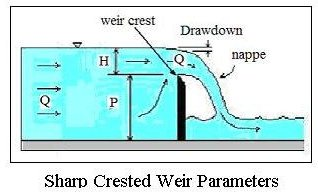The Excel templates in this article will calculate the water flow rate over a rectangular weir based on input information about the weir and open channel geometry and the measured head over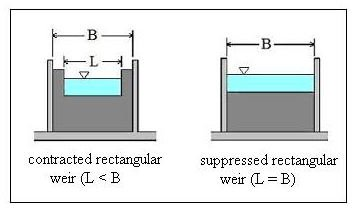the weir. The diagram at the left shows a longitudinal view of a sharp crested, rectangular weir with some of the parameters. The diagram at the right shows the difference between a suppressed rectangular weir (weir length = channel width) and a contracted rectangular weir (weir length < channel width).

The next two sections will present and discuss Excel templates that have the weir equations entered in as Excel formulas to make calculations for a suppressed rectangular weir and for a contracted rectangular weir.

For more background information about rectangular sharp crested weirs for measuring open channel flow rate, see the article, "Open Channel Flow Measurement 5: the Rectangular Weir."

## Suppressed Rectangular Weir Flow Calculator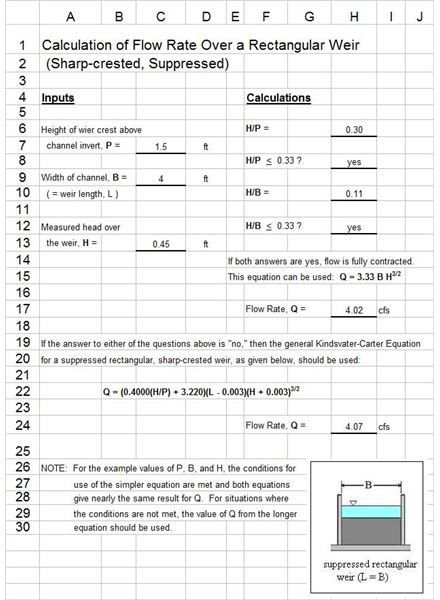The Excel template shown at the left will calculate the water flow rate over a suppressed rectangular weir. Three input values are needed: the height of the weir crest above the channel invert, P; the width of the channel, B (which is also equal to the weir length L); and the measured head over the weir, H. With these three input values, the Excel formulas will calculate H/P and H/B. If both of these are less than 0.33, then the equation, Q = 3.33 B H3/2, can be used to calculate the weir flow, Q.

If either H/P or H/B is greater than 0.33, then the Kindvater-Carter equation should be used as follows:

Q = (0.4000)(H/P) + 3.220)(L – 0.003)(H + 0.003)3/2.

The Excel spreadsheet template is set up to calculate Q using both equations. If H/P < 0.33 and H/B < 0.33, as in the example spreadsheet, then the two Q values will be nearly the same. If either H/P > 0.33 and/or H/B > 0.33, then there will be a greater difference between the two Q values and Q from the Kindsvater-Carter equation should be used.

## Contracted Rectangular Weir Flow Calculator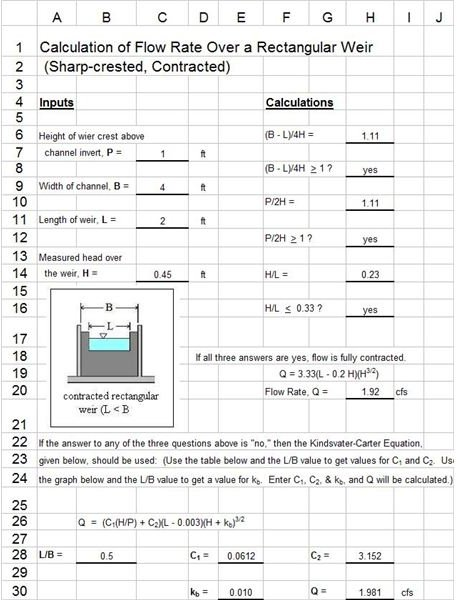The first page of the Excel template for contracted rectangular weir flow calculations is shown at the left and a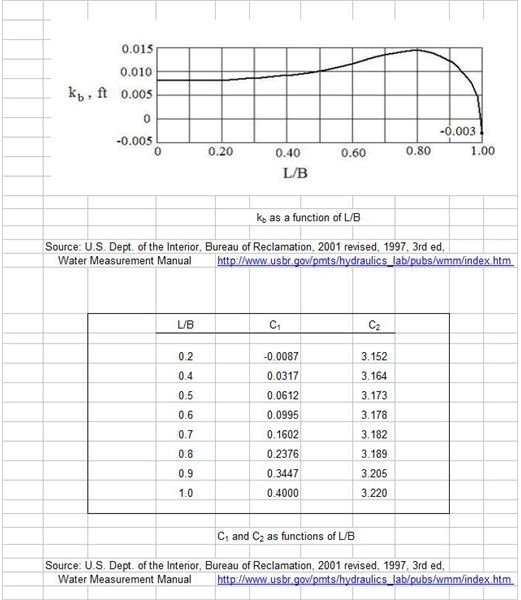second page is shown at the right. For a contracted rectangular weir, L < B, so in addition to the three input values needed for the suppressed rectangular weir (P, B, and H), a value for the weir length, L, must be entered.

The Excel formulas in this spreadsheet template will then calculate (B – L)/4H, P/H, and H/L. If (B -L)/4H and P/H are greater than 1 and H/L < 0.33, then the simpler equation, Q = 3.33(L – 0.2H)H3/2, can be used.

If any of the three conditions are not met, then the equation to use is:

Q = (C1(H/P) + C2)(L – 0.003)(H + kb)3/2

If any of the three conditions are not met, you will need to use the value of L/B calculated by the spreadsheet, together with the graph and table on the second page, to obtain values for C1, C2, and kb, and enter them in the indicated cells. The Excel formula built in the spreadsheet template will then calculate Q using the above equation.

## References and Image Credits

References for further information:

1. U.S. Dept. of the Interior, Bureau of Reclamation, 2001 revised, 1997 third edition, Water Measurement Manual.

2. Bengtson, Harlan H., Open Channel Flow III – Sharp Crested Weirs, an online continuing education course for PDH credit.

3. Munson, B. R., Young, D. F., & Okiishi, T. H., Fundamentals of Fluid Mechanics, 4th Ed., New York: John Wiley and Sons, Inc, 2002.

Images were all prepared by H. Bengtson

## This post is part of the series: Excel Spreadsheets for Open Channel Flow Measurement Calculations

These contain Excel spreadsheets that can be downloaded for open channel flow measurement devices, such as V-notch weirs and rectangular weirs. The Excel spreadsheets can be used as a weir flow calculated in each case.